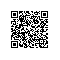# 极速理解设计模式系列：24.解释器模式(Interpreter Pattern)

场景(Context)：解释器的全局信息

抽象表达式(Component)：定义一个接口来解释操作

终结符表达式(TerminalExpression)：直接跳过步骤，不用解释语句

非终结符表达式(NonterminalExpression)：根据规则实现解释操作

客户端(Client):调用解释器，对语句进行解释。下面我们在控制台程序去演示一下如何使用Interpreter Pattern：

一、 场景(Context)

1. //场景(Context)
2.   class Context
3.   {
4.
5.       public Context(string input)
6.       {
7.           this.Input = input;
8.       }
9.
10.       /// <summary>
11.       /// 输入参数
12.       /// </summary>
13.       public string Input { get; set; }
14.
15.       /// <summary>
16.       /// 输出参数
17.       /// </summary>
18.       public int Output { get; set; }
19.
20.       /// <summary>
21.       /// 是否是十六进制 如果是转为十进制,否则不转
22.       /// </summary>
23.       public bool Status { get; set; }
24.   }

二、抽象表达式(Component)

1. //抽象表达式类(AbstractExpression)
2. abstract class Expression
3.     public virtual void Interpret(Context context)
4.     {
5.         if (context.Input.Length == 0)
6.             return
7.
8.         int multiresult = Multiplier(context);
9.         if (multiresult == 0)
10.             return
11.
12.         if(context.Input.StartsWith("F"))
13.         {
14.             context.Output += (15 * multiresult);
15.             context.Input=context.Input.Substring(1);
16.         }
17.         else if (context.Input.StartsWith("F"))
18.         {
19.             context.Output += (15 * multiresult);
20.             context.Input = context.Input.Substring(1);
21.         }
22.         else if (context.Input.StartsWith("E"))
23.         {
24.             context.Output += (14 * multiresult);
25.             context.Input = context.Input.Substring(1);
26.         }
27.         else if (context.Input.StartsWith("D"))
28.         {
29.             context.Output += (13 * multiresult);
30.             context.Input = context.Input.Substring(1);
31.         }
32.         else if (context.Input.StartsWith("C"))
33.         {
34.             context.Output += (12 * multiresult);
35.             context.Input = context.Input.Substring(1);
36.         }
37.         else if (context.Input.StartsWith("B"))
38.         {
39.             context.Output += (11 * multiresult);
40.             context.Input = context.Input.Substring(1);
41.         }
42.         else if (context.Input.StartsWith("A"))
43.         {
44.             context.Output += (10 * multiresult);
45.             context.Input = context.Input.Substring(1);
46.         }
47.         else
48.         {
49.             context.Output += (int.Parse(context.Input.Substring(0, 1)) * multiresult);
50.             context.Input = context.Input.Substring(1);
51.         }
52.     }
53.
54.     //该位置需要做的乘法值
55.     public abstract int Multiplier(Context context);

三、终结符表达式(TerminalExpression)

1. //终结符表达式(TerminalExpression)
2.  class NumterminalExp : Expression
3.  {
4.      public override void Interpret(Context context)
5.      {
6.          if (context.Input.StartsWith("0X"))
7.          {
8.              context.Input = context.Input.Substring(2);
9.              context.Status = true
10.          }
11.          else
12.          {
13.              context.Output = int.Parse(context.Input);
14.              context.Status = false
15.              return
16.          }
17.      }
18.      public override int Multiplier(Context context)
19.      {
20.          return 1;
21.      }
22.  }

四、非终结符表达式(NonterminalExpression)

1. //非终结符表达式(NonterminalExpression)  千位计算
2. class ThousandExp : Expression
3.     public override int Multiplier(Context context)
4.     {
5.         if (context.Input.Length == 4&&context.Status)
6.             return 16 * 16 * 16;
7.         else
8.             return 0;
9.     }
10.
11. //非终结符表达式(NonterminalExpression)  百位计算
12. class HundredExp : Expression
13.     public override int Multiplier(Context context)
14.     {
15.         if (context.Input.Length == 3 && context.Status)
16.             return 16 * 16;
17.         else
18.             return 0;
19.     }
20.
21. //非终结符表达式(NonterminalExpression)  十位计算
22. class TenExp : Expression
23.     public override int Multiplier(Context context)
24.     {
25.         if (context.Input.Length == 2 && context.Status)
26.             return 16;
27.         else
28.             return 0;
29.     }
30.
31. //非终结符表达式(NonterminalExpression)  个位计算
32. class OneExp : Expression
33.     public override int Multiplier(Context context)
34.     {
35.         if (context.Input.Length == 1 && context.Status)
36.             return 1;
37.         else
38.             return 0;
39.     }

五、客户端(Client)

1. //客户端(Client)
2. class Program
3.     static void Main(string[] args)
4.     {
5.         string input = "0XA321"
6.
7.         Context context = new Context(input.ToUpper());
8.
9.         List<Expression> expTree = new List<Expression>();
15.
16.         foreach (Expression exp in expTree)
17.         {
18.             exp.Interpret(context);
19.         }
20.         Console.WriteLine("十六进制数{0}转换为十进制数{1}", input, context.Output);
22.     }

如需源码请点击 InterpreterPattern.rar 下载使用钉钉扫一扫加入圈子
+ 订阅